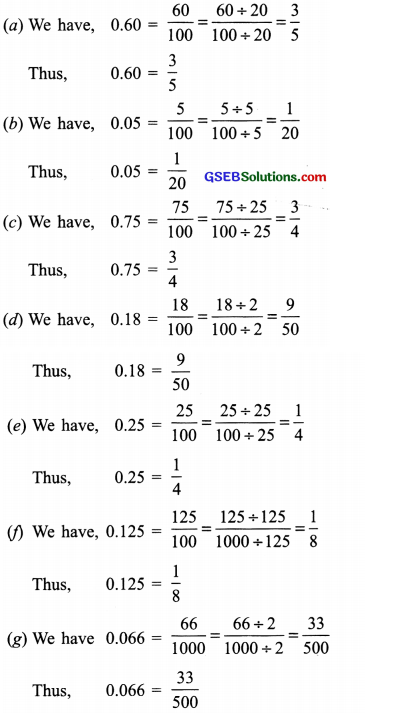# GSEB Solutions Class 6 Maths Chapter 8 Decimals Ex 8.2

Gujarat Board GSEB Textbook Solutions Class 6 Maths Chapter 8 Decimals Ex 8.2 Textbook Questions and Answers.

## Gujarat Board Textbook Solutions Class 6 Maths Chapter 8 Decimals Ex 8.2

Question 1.
Complete the table with the help of these boxes and use decimals to write the number: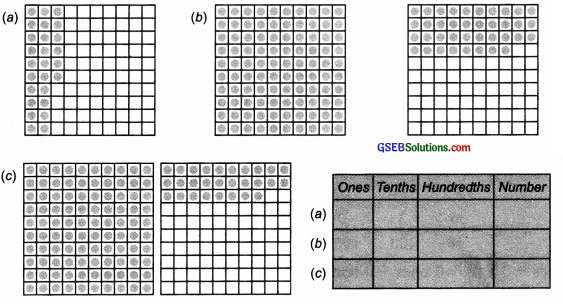Solution:
Since in figure:
(a) 26 small parts out of 100 are shaded
∴ The decimal 0.26 is represented.

(b) 100 parts + 38 parts are shaded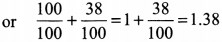1.38 is represented by the shaded portion.

(c) 100 parts + 28 parts are shaded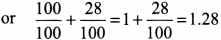1.28 is represented by the shaded portion. Therefore, the table is compiled as: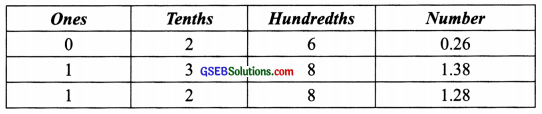Question 2.
Write the numbers given in the following place value table in decimal form.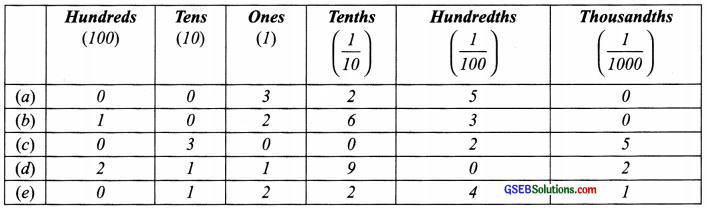Solution: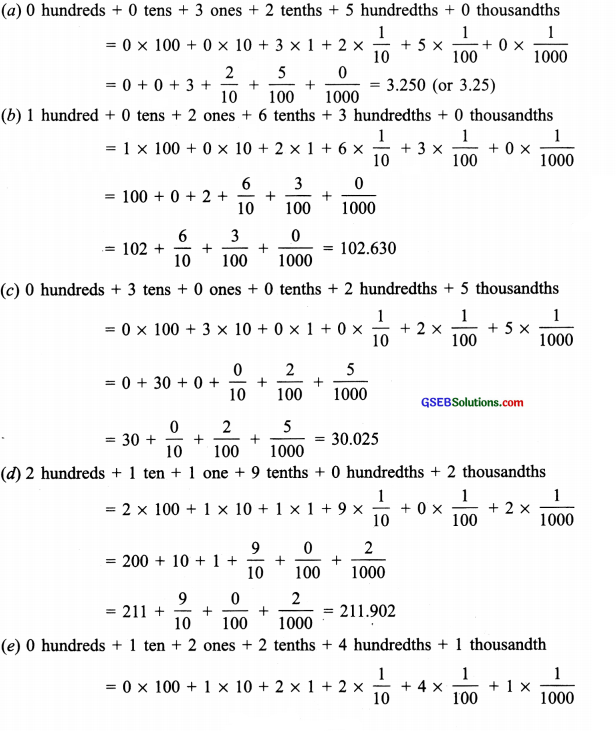Question 3.
Write the following decimals in the place value table.
(a) 0.29
(b) 2.08
(c) 19.60
(d) 148.32
(e) 200.812
Solution:
(a) 0.29 = 0 × 1 + $$\frac { 2 }{ 10 }$$ + $$\frac { 9 }{ 100 }$$

(b) 2.08 = 2 × 1 + $$\frac { 0 }{ 10 }$$ + $$\frac { 8 }{ 100 }$$

(c) 19.60 = 1 × 10 + 9 × 1 + $$\frac { 6 }{ 10 }$$ + $$\frac { 0 }{ 100 }$$

(d) 148.32 = 1 × 100 + 4 × 10 + 8 × 1 + $$\frac { 3 }{ 10 }$$ + $$\frac { 2 }{ 100 }$$

(e) 200.812 = 2 × 100 + 0 × 10 + 0 × 1 + $$\frac { 8 }{ 10 }$$ + $$\frac { 1 }{ 100 }$$ + $$\frac { 2 }{ 1000 }$$
Now, we can write the given decimals in the place value table as: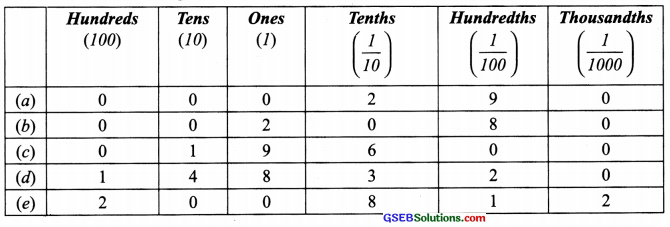Question 4.
Write each of the following as decimals.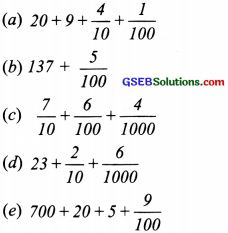Solution: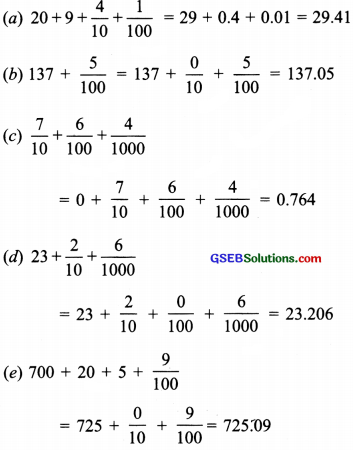Question 5.
Write each of the following decimals in words.
(a) 0.03
(b) 1.20
(c) 108.56
(d) 10.07
(e) 0.032
(f) 5.008
Solution:
(a) Zero point zero three
(b) One point two zero
(c) One hundred eight point five six
(d) Ten point zero seven
(e) Zero point zero three two
(f) Five-point zero zero eightQuestion 6.
Between which two numbers in tenths place on the number line does each of the given numbers lies?
(a) 0.06
(b) 0.45
(c) 0.19
(d) 0.66
(e) 0.92
(f) 0.57
Solution:
(a) 0.06 lies between 0 and 0.1
(b) 0.45 lies between 0.4 and 0.5
(c) 0.19 lies between 0.1 and 0.2
(d) 0.66 lies between 0.6 and 0.7
(e) 0.92 lies between 0.9 and 1.0
(f) 0.57 lies between 0.5 and 0.6Question 7.
Write as fractions in lowest terms.
(a) 0.60
(b) 0.05
(c) 0.75
(d) 0.18
(e) 0.25
(f) 0.125
(g) 0.066
Solution: